Cengiz Zopluoglu (University of Oregon)
11/23/2022

[Updated: Sun, Nov 27, 2022 - 14:16:59 ]

In Bagged Trees or Random Forests models, the trees are developed independently by taking a random sample of rows and columns from the dataset. The main difference between Gradient Boosting Trees and Bagged Trees or Random Forests is that the trees are developed sequentially, and each tree model is built upon the errors of the previous tree models. The sequential process of model building and predictions in gradient-boosted trees can be conceptually demonstrated below.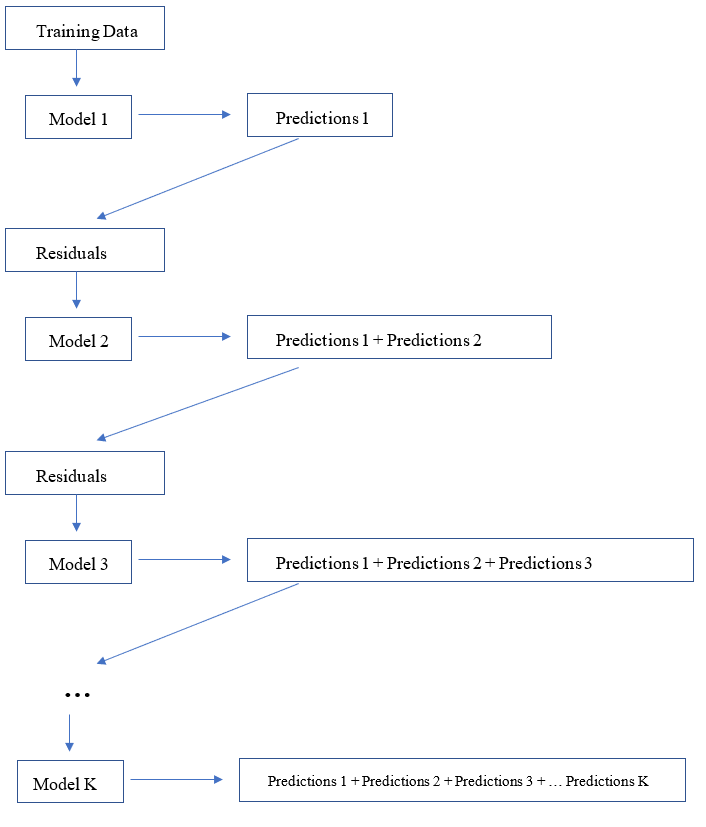# 1. Understanding the Machinery of GBM with Sequential Development of Trees from Residuals

Let’s try to implement this idea in a toy dataset we use to predict a readability score from the number of sentences.

readability_sub <- read.csv('./data/readability_sub.csv',header=TRUE)

readability_sub[,c('V220','V166','target')]
          V220        V166      target
1  -0.13908258  0.19028091 -2.06282395
2   0.21764143  0.07101288  0.58258607
3   0.05812133  0.03993277 -1.65313060
4   0.02526429  0.18845809 -0.87390681
5   0.22430885  0.06200715 -1.74049148
6  -0.07795373  0.10754109 -3.63993555
7   0.43400714  0.12202360 -0.62284268
8  -0.24364550  0.02454670 -0.34426981
9   0.15893717  0.10422343 -1.12298826
10  0.14496475  0.02339597 -0.99857142
11  0.34222975  0.22065343 -0.87656742
12  0.25219145  0.10865010 -0.03304643
13  0.03532625  0.07549474 -0.49529863
14  0.36410633  0.18675801  0.12453660
15  0.29988593  0.11618323  0.09678258
16  0.19837037  0.08272671  0.38422270
17  0.07807041  0.10235218 -0.58143038
18  0.07935690  0.11618605 -0.34324576
19  0.57000953 -0.02385423 -0.39054205
20  0.34523284  0.09299514 -0.67548411

## Iteration 0

We start with a simple model that uses the average target outcome to predict the readability for all observations in this toy dataset. We calculate the predictions and residuals from this initial intercept-only model.

readability_sub$pred0 <- mean(readability_sub$target)
readability_sub$res0 <- readability_sub$target - readability_sub$pred round(readability_sub[,c('V166','V220','target','pred0','res0')],3)  V166 V220 target pred0 res0 1 0.190 -0.139 -2.063 -0.763 -1.300 2 0.071 0.218 0.583 -0.763 1.346 3 0.040 0.058 -1.653 -0.763 -0.890 4 0.188 0.025 -0.874 -0.763 -0.111 5 0.062 0.224 -1.740 -0.763 -0.977 6 0.108 -0.078 -3.640 -0.763 -2.877 7 0.122 0.434 -0.623 -0.763 0.140 8 0.025 -0.244 -0.344 -0.763 0.419 9 0.104 0.159 -1.123 -0.763 -0.360 10 0.023 0.145 -0.999 -0.763 -0.235 11 0.221 0.342 -0.877 -0.763 -0.113 12 0.109 0.252 -0.033 -0.763 0.730 13 0.075 0.035 -0.495 -0.763 0.268 14 0.187 0.364 0.125 -0.763 0.888 15 0.116 0.300 0.097 -0.763 0.860 16 0.083 0.198 0.384 -0.763 1.148 17 0.102 0.078 -0.581 -0.763 0.182 18 0.116 0.079 -0.343 -0.763 0.420 19 -0.024 0.570 -0.391 -0.763 0.373 20 0.093 0.345 -0.675 -0.763 0.088 # SSE at the end of Iteration 0 sum(readability_sub$res0^2)
 17.73309

## Iteration 1

Now, we fit a tree model to predict the residuals of Iteration 0 from the two predictors (Features V220 and V166). Notice that we fix the value of specific parameters while fitting the tree model (e.g., cp, minsplit,maxdepth).

require(rpart)
require(rattle)

model1 <- rpart(formula = res0 ~ V166 + V220,
method  = "anova",
control = list(minsplit=2,
cp=0,
minbucket = 2,
maxdepth = 2)
)

fancyRpartPlot(model1,type=2,sub='')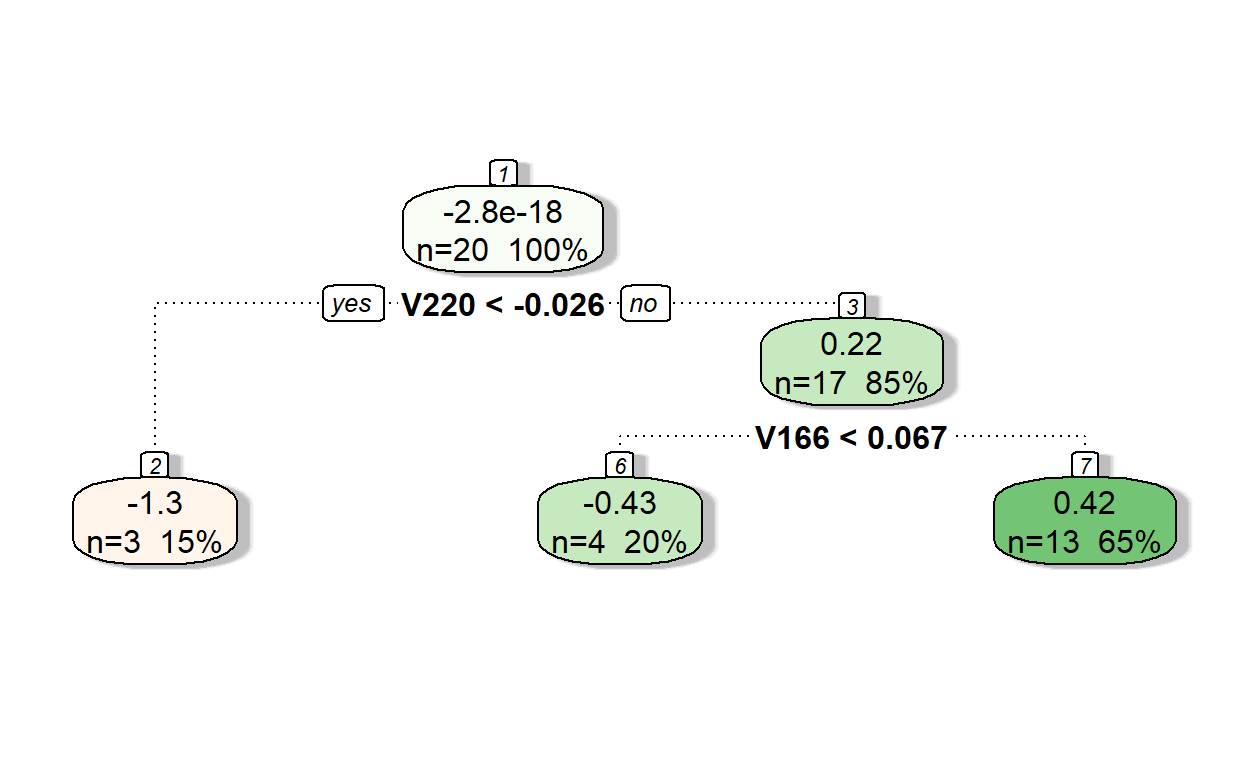Let’s see the predictions of residuals from Model 1.

pr.res <- predict(model1, readability_sub)
pr.res 
         1          2          3          4          5          6
-1.2523541  0.4220391 -0.4323615  0.4220391 -0.4323615 -1.2523541
7          8          9         10         11         12
0.4220391 -1.2523541  0.4220391 -0.4323615  0.4220391  0.4220391
13         14         15         16         17         18
0.4220391  0.4220391  0.4220391  0.4220391  0.4220391  0.4220391
19         20
-0.4323615  0.4220391 

Now, let’s add the predicted residuals from Iteration 1 to the predictions from Iteration 0 to obtain the new predictions.

readability_sub$pred1 <- readability_sub$pred0 + pr.res
readability_sub$res1 <- readability_sub$target - readability_sub$pred1 round(readability_sub[,c('V166','V220','target','pred0','res0','pred1','res1')],3)  V166 V220 target pred0 res0 pred1 res1 1 0.190 -0.139 -2.063 -0.763 -1.300 -2.016 -0.047 2 0.071 0.218 0.583 -0.763 1.346 -0.341 0.924 3 0.040 0.058 -1.653 -0.763 -0.890 -1.196 -0.457 4 0.188 0.025 -0.874 -0.763 -0.111 -0.341 -0.533 5 0.062 0.224 -1.740 -0.763 -0.977 -1.196 -0.545 6 0.108 -0.078 -3.640 -0.763 -2.877 -2.016 -1.624 7 0.122 0.434 -0.623 -0.763 0.140 -0.341 -0.282 8 0.025 -0.244 -0.344 -0.763 0.419 -2.016 1.671 9 0.104 0.159 -1.123 -0.763 -0.360 -0.341 -0.782 10 0.023 0.145 -0.999 -0.763 -0.235 -1.196 0.197 11 0.221 0.342 -0.877 -0.763 -0.113 -0.341 -0.535 12 0.109 0.252 -0.033 -0.763 0.730 -0.341 0.308 13 0.075 0.035 -0.495 -0.763 0.268 -0.341 -0.154 14 0.187 0.364 0.125 -0.763 0.888 -0.341 0.466 15 0.116 0.300 0.097 -0.763 0.860 -0.341 0.438 16 0.083 0.198 0.384 -0.763 1.148 -0.341 0.726 17 0.102 0.078 -0.581 -0.763 0.182 -0.341 -0.240 18 0.116 0.079 -0.343 -0.763 0.420 -0.341 -0.002 19 -0.024 0.570 -0.391 -0.763 0.373 -1.196 0.805 20 0.093 0.345 -0.675 -0.763 0.088 -0.341 -0.334 # SSE at the end of Iteration 1 sum(readability_sub$res1^2)
 9.964654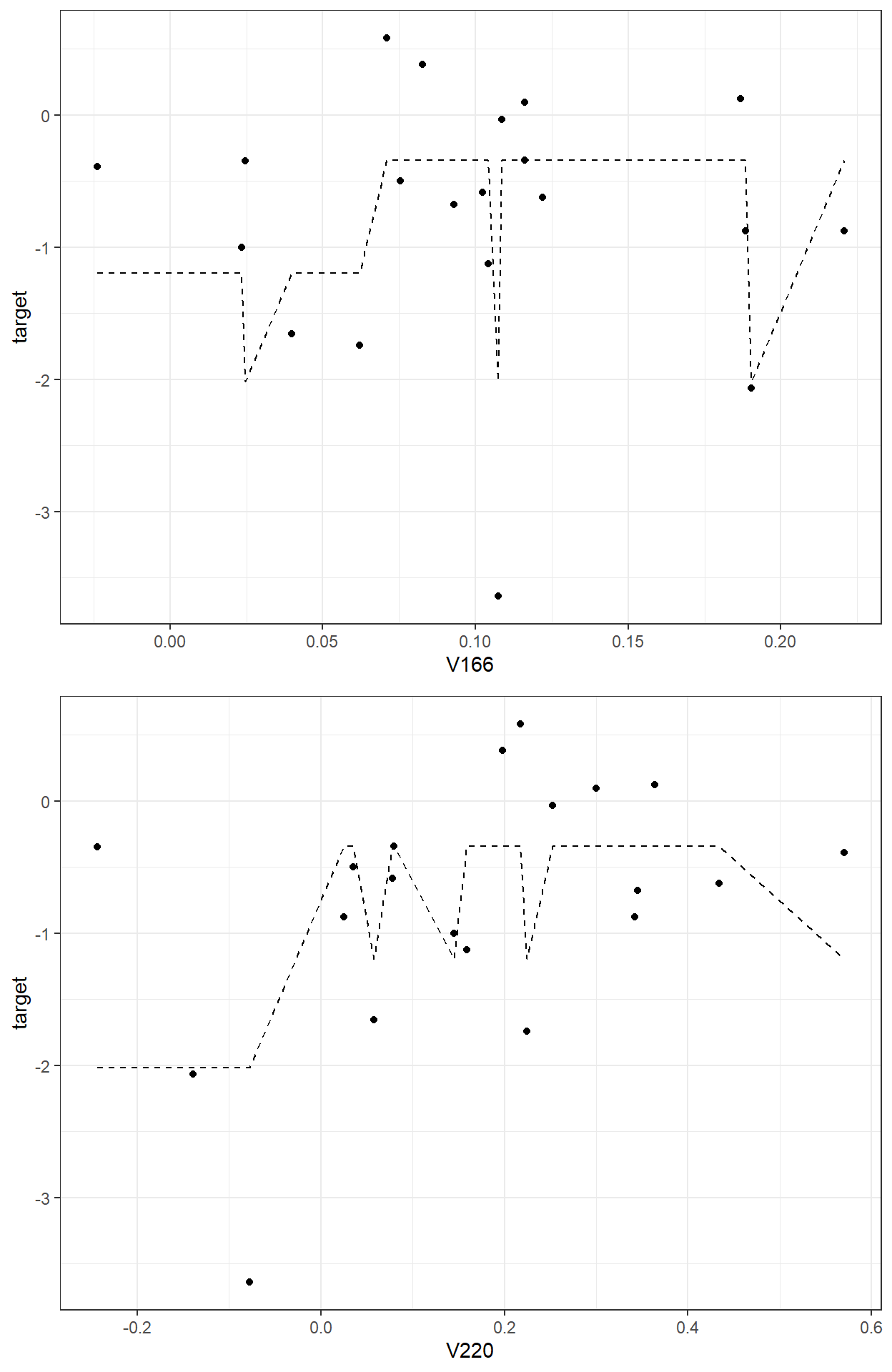## Iteration 2

We repeat Iteration 1, but the only difference is that we now fit a tree model to predict the residuals at the end of Iteration 1.

model2 <- rpart(formula = res1 ~  V166 + V220,
method  = "anova",
control = list(minsplit=2,
cp=0,
minbucket = 2,
maxdepth = 2)
)

fancyRpartPlot(model2,type=2,sub='')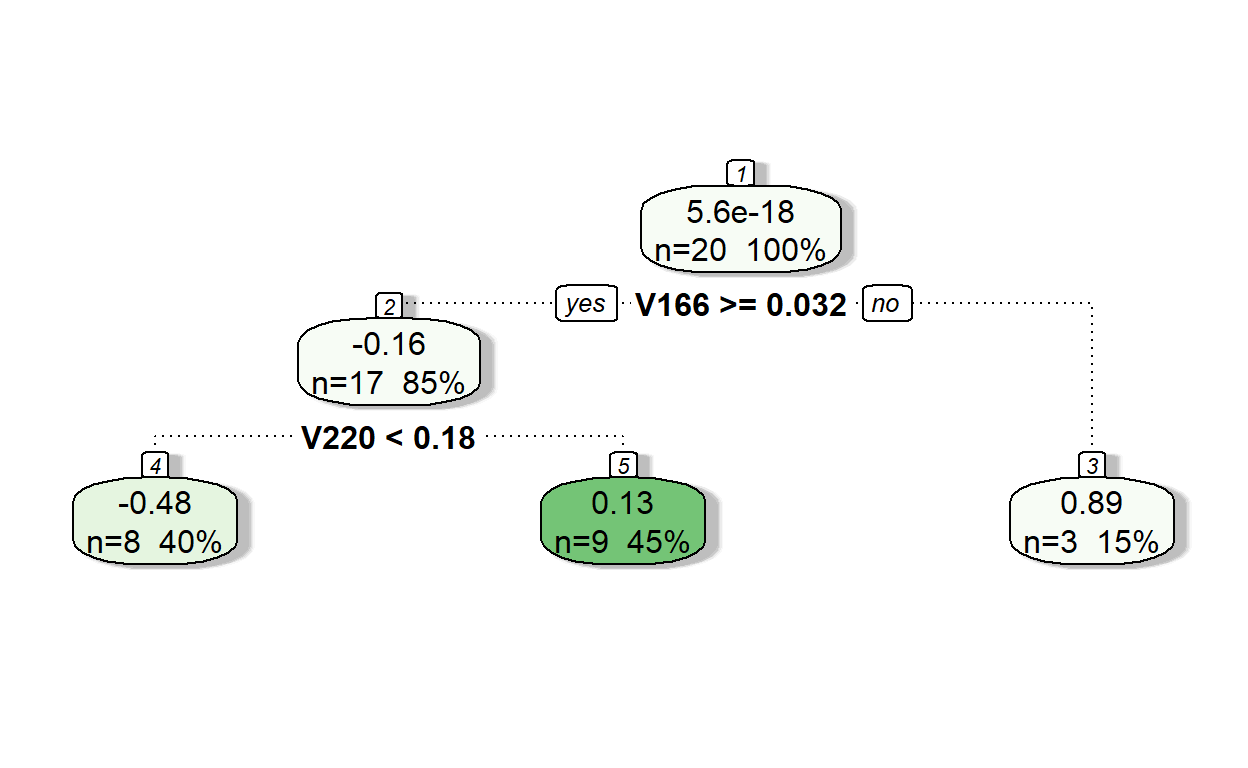Let’s see the predictions of residuals from Model 2.

pr.res <- predict(model2, readability_sub)
pr.res
         1          2          3          4          5          6
-0.4799134  0.1295162 -0.4799134 -0.4799134  0.1295162 -0.4799134
7          8          9         10         11         12
0.1295162  0.8912203 -0.4799134  0.8912203  0.1295162  0.1295162
13         14         15         16         17         18
-0.4799134  0.1295162  0.1295162  0.1295162 -0.4799134 -0.4799134
19         20
0.8912203  0.1295162 

Now, add the predicted residuals from Iteration 2 to the predictions from Iteration 1 to obtain the new predictions.

readability_sub$pred2 <- readability_sub$pred1 + pr.res
readability_sub$res2 <- readability_sub$target - readability_sub$pred2 round(readability_sub[,c('V166','V220','target', 'pred0','res0','pred1','res1', 'pred2','res2')],3)  V166 V220 target pred0 res0 pred1 res1 pred2 res2 1 0.190 -0.139 -2.063 -0.763 -1.300 -2.016 -0.047 -2.496 0.433 2 0.071 0.218 0.583 -0.763 1.346 -0.341 0.924 -0.212 0.794 3 0.040 0.058 -1.653 -0.763 -0.890 -1.196 -0.457 -1.676 0.022 4 0.188 0.025 -0.874 -0.763 -0.111 -0.341 -0.533 -0.821 -0.053 5 0.062 0.224 -1.740 -0.763 -0.977 -1.196 -0.545 -1.066 -0.674 6 0.108 -0.078 -3.640 -0.763 -2.877 -2.016 -1.624 -2.496 -1.144 7 0.122 0.434 -0.623 -0.763 0.140 -0.341 -0.282 -0.212 -0.411 8 0.025 -0.244 -0.344 -0.763 0.419 -2.016 1.671 -1.124 0.780 9 0.104 0.159 -1.123 -0.763 -0.360 -0.341 -0.782 -0.821 -0.302 10 0.023 0.145 -0.999 -0.763 -0.235 -1.196 0.197 -0.304 -0.694 11 0.221 0.342 -0.877 -0.763 -0.113 -0.341 -0.535 -0.212 -0.665 12 0.109 0.252 -0.033 -0.763 0.730 -0.341 0.308 -0.212 0.179 13 0.075 0.035 -0.495 -0.763 0.268 -0.341 -0.154 -0.821 0.326 14 0.187 0.364 0.125 -0.763 0.888 -0.341 0.466 -0.212 0.336 15 0.116 0.300 0.097 -0.763 0.860 -0.341 0.438 -0.212 0.309 16 0.083 0.198 0.384 -0.763 1.148 -0.341 0.726 -0.212 0.596 17 0.102 0.078 -0.581 -0.763 0.182 -0.341 -0.240 -0.821 0.240 18 0.116 0.079 -0.343 -0.763 0.420 -0.341 -0.002 -0.821 0.478 19 -0.024 0.570 -0.391 -0.763 0.373 -1.196 0.805 -0.304 -0.086 20 0.093 0.345 -0.675 -0.763 0.088 -0.341 -0.334 -0.212 -0.464 # SSE at the end of Iteration 2 sum(readability_sub$res2^2)
 5.588329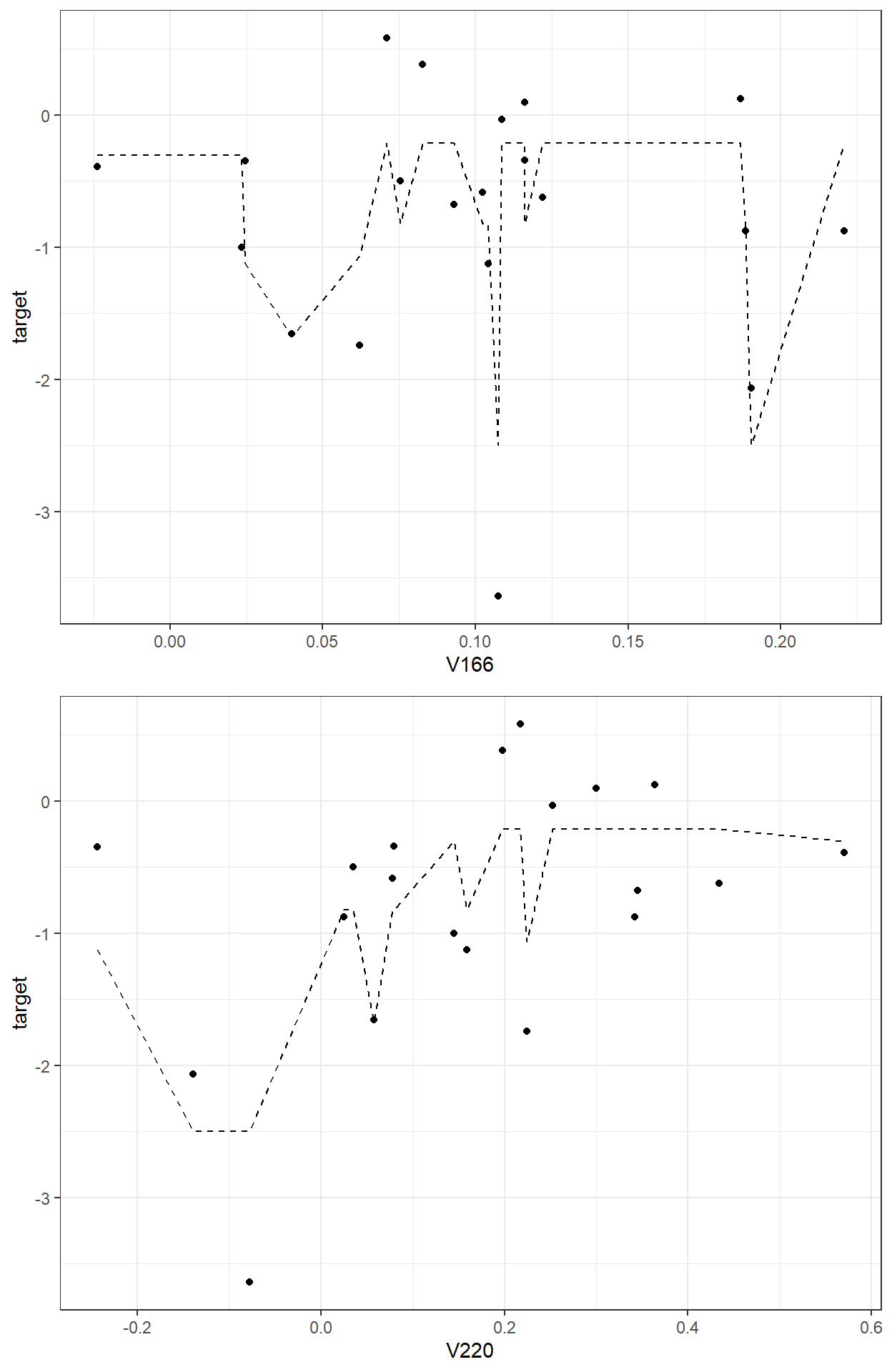We can keep iterating and add tree models as long as we find a tree model that improves our predictions (minimizing SSE).

## 2. A more formal introduction of Gradient Boosting Trees

Let $$\mathbf{x}_i = (x_{i1},x_{i2},x_{i3},...,x_{ij})$$ represent a vector of observed values for the $$i^{th}$$ observation on $$j$$ predictor variables, and $$y_i$$ is the value of the target outcome for the $$i^{th}$$ observation. A gradient-boosted tree model is an ensemble of $$T$$ different tree models sequentially developed, and the final prediction of the outcome is obtained by using an additive function as

$\hat{y_i} = \sum_{t=1}^{T}f_t(\mathbf{x}_i),$

where $$f_t$$ is a tree model obtained at Iteration $$t$$ from the residuals at Iteration $$t-1$$.

The algorithm optimizes an objective function $$\mathfrak{L}(\mathbf{y},\mathbf{\hat{y}})$$ in an additive manner. This objective loss function can be defined as the sum of squared errors when the outcome is continuous or logistic loss when the outcome is categorical.

The algorithm starts with a constant prediction. For instance, we start with the average outcome in the above example. Then, a new tree model that minimizes the objective loss function is searched and added at each iteration.

$\hat{y}_{i}^{(0)} = \bar{y}$ $\hat{y}_{i}^{(1)} = \hat{y}_{i}^{(0)} + \alpha f_1(\mathbf{x}_i)$

$\hat{y}_{i}^{(2)} = \hat{y}_{i}^{(1)} + \alpha f_2(\mathbf{x}_i)$ $.$ $.$ $.$ $\hat{y}_{i}^{(t)} = \hat{y}_{i}^{(t-1)} + \alpha f_t(\mathbf{x}_i)$

Notice that I added a multiplier, $$\alpha$$ while adding our predictions at each iteration. In the above example, we fixed this multiplier to 1, $$\alpha = 1$$, as we added a whole new prediction to the previous prediction. This multiplier in machine learning literature is called the learning rate. We could also choose to add only a fraction of new predictions (e.g., $$\alpha = 0.1,0.05,0.01,0.001$$) at each iteration.

The smaller the learning rate, the more iterations (more tree models) we will need to achieve the same level of performance. So, the number of iterations (number of tree models, $$T$$) and the learning rate ($$\alpha$$) play in tandem. These two parameters are known as the boosting hyperparameters and need to be tuned.

Think about choosing a learning rate as choosing your speed on a highway and number of trees as the time it takes to arrive at your destination. Suppose you are traveling from Eugene to Portland on I-5. If you drive 40 miles/hour, you are less likely to involve in an accident because you are more aware of your surroundings, but it will take 3-4 hours to arrive at your destination. If you are 200 miles/hour, it will only take an hour to arrive at your destination, assuming you will not have an accident on the way (which is very likely). So, you try to find a speed level that is fast enough to arrive at your destination and safe enough not to have an accident.

TECHNICAL NOTE

Why do people call it Gradient Boosting? It turns out that the updates at each iteration based on the residuals from a previous model are related to the concept of negative gradient (first derivative) of the objective loss function with respect to the predicted values from the previous step.

$-g_i^t = -\frac{\partial \mathfrak{L}(y_i,\hat{y}_i^{t-1})}{\partial \hat{y}_i^{t-1}} = \hat{y}_{i}^{(t)} - \hat{y}_{i}^{(t-1)}$

The general logic of gradient boosting works as

• take a differentiable loss function, $$\mathfrak{L}(\mathbf{y},\mathbf{\hat{y}})$$, that summarizes the distance between observed and predicted values,

• start with an initial model to obtain initial predictions, $$f_0(\mathbf{x}_i)$$,

• iterate until termination:

• calculate the negative gradients of the loss function with respect to predictions from the previous step

• fit a tree model to the negative gradients

• update the predictions (with a multiplier, a.k.a learning rate).

Most software uses mathematical approximations and computational hocus pocus to do these computations for faster implementation.

# 3. Fitting Gradient Boosting Trees using the gbm package

The gradient boosting trees can be fitted using the gbm function from the gbm package. The code below tries to replicate our example above using the toy dataset.

require(gbm)

gbm.model <-   gbm(formula           = target ~ V166 + V220,
distribution       = 'gaussian',
n.trees           = 2,
shrinkage         = 1,
interaction.depth = 2,
n.minobsinnode    = 2,
bag.fraction      = 1,
cv.folds          = 0,
n.cores           = 1)

Model and Data:

• formula, a description of the outcome and predictive variables in the model using column names

• data, the name of the data object to look for the variables in the formula statement

• distribution, a character to specify the type of objective loss function to optimize. ‘gaussian’ is typically used for continuous outcomes(minimize the squared error), and ‘bernoulli’ is typically used for the binary outcomes (minimizes the logistic loss)

Hyperparameters:

• n.trees, number of trees to fit (the number of iterations)

• shrinkage, learning rate.

• interaction.depth, the maximum depth of each tree developed at each iteration

• n.minobsinnode, the minimum number of observations in each terminal note of tree models at each iteration

• bag.fraction, the proportion of observations to be randomly selected for developing a new tree at each iteration.

In Gradient Boosting Trees, we use all observations (100% of rows) when we develop a new tree model at each iteration. So, we can set bag.fraction=1, and gbm fits a gradient boosting tree model. On the other hand, adding a random component may help yield better performance. You can think about this as a marriage of Bagging and Boosting. So, we may want to take a random sample of observations to develop a tree model at each iteration. For instance, if you set bag.fraction=.9, the algorithm will randomly sample 90% of the observations at each iteration before fitting the new tree model to residuals from the previous step. When bag.fraction is lower than 1, this is called Stochastic Gradient Boosting Trees. bag.fraction can also be considered a hyperparameter to tune by trying different values to find an optimal value, or it can be fixed to a certain number.

Cross-validation:

• cv.folds, number of cross-validation folds to perform.

Parallel Processing:

• n.cores, the number of CPU cores to use.
# Obtain predictions from the model

predict(gbm.model)
  -2.4955898 -0.2117671 -1.6755972 -0.8211966 -1.0661677 -2.4955898
 -0.2117671 -1.1244561 -0.8211966 -0.3044636 -0.2117671 -0.2117671
 -0.8211966 -0.2117671 -0.2117671 -0.2117671 -0.8211966 -0.8211966
 -0.3044636 -0.2117671
# Plot the final model

plot(gbm.model,i.var = 1)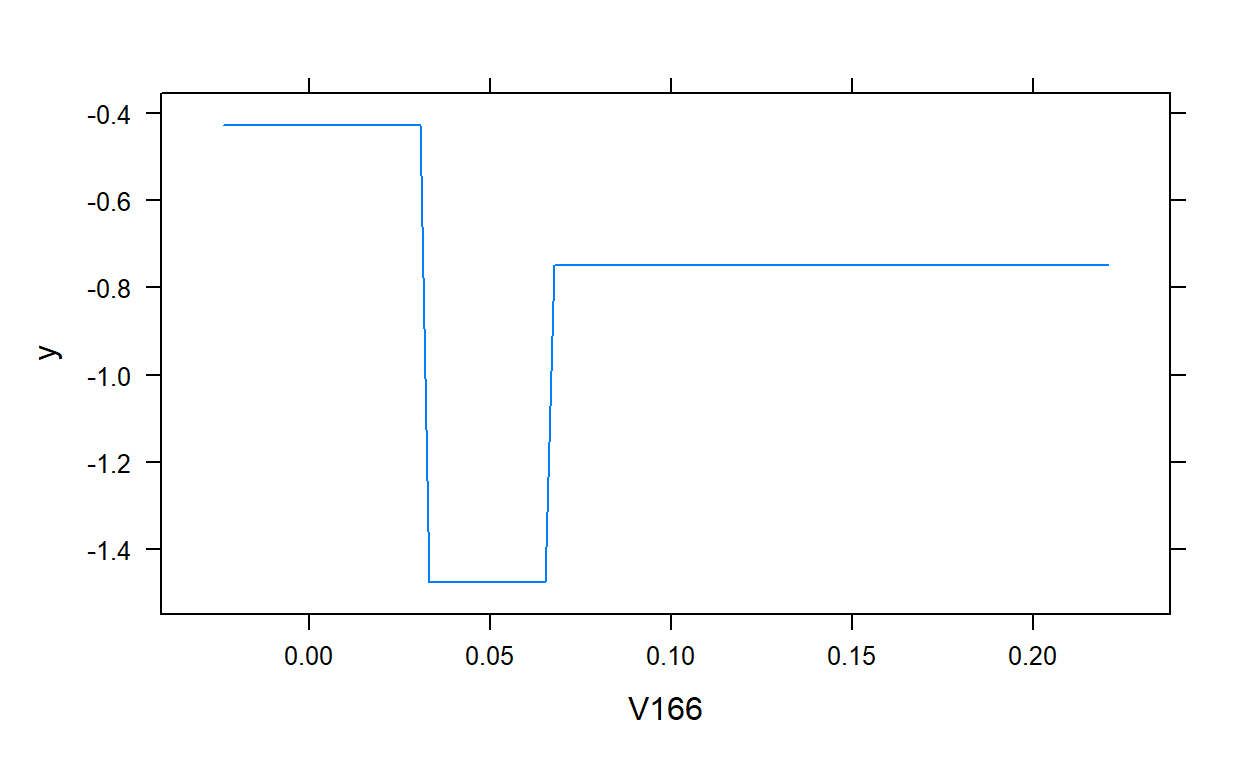plot(gbm.model,i.var = 2)# 4. Fitting Gradient Boosting Trees using the caret package and Hyperparameter Tuning

The gbm algorithm is available in the caret package. Let’s check the hyperparameters available to tune.

require(caret)

getModelInfo()$gbm$parameters
          parameter   class                   label
1           n.trees numeric   # Boosting Iterations
2 interaction.depth numeric          Max Tree Depth
3         shrinkage numeric               Shrinkage
4    n.minobsinnode numeric Min. Terminal Node Size

The four most critical parameters are all available to tune. It is very challenging to find the best combination of values for all these four hyperparameters unless you implement a full grid search which may take a very long time. You may apply a general sub-optimal strategy to tune the hyperparameters step by step, either in pairs or one by one. Below is one way to implement such a strategy:

1. Fix the interaction.depth and n.minobsinnode to a certain value (e.g., interaction.depth = 5, n.minobsinnode = 10),

2. Pick a small value of learning rate (shrinkage), such as 0.05 or 0.1,

3. Do a grid search and find the optimal number of trees (n.trees) using the fixed values at #1 and #2,

4. Fix the n.trees at its optimal value from #3, keep shrinkage the same as in #2, and do a two-dimensional grid search for interaction.depth and n.minobsinnode and find the optimal number of depth and minimum observation in a terminal node,

5. Fix the interaction.depth and n.minobsinnode’at their optimal values from #4, lower the learning rate and increase the number of trees to see if the model performance can be further improved.

6. Fix interaction.depth,n.minobsinnode, shrinkage, and n.trees at their optimal values from previus steps, and do a grid search for bag.fraction.

You will find an interactive app you can play at the link below to understand the dynamics among these hyperparameters and optimize them in toy examples.

First, we import and prepare data for modeling. Then, we split the data into training and test pieces.

require(recipes)
require(caret)

# Import the dataset

# Write the recipe

roles = c(rep('predictor',768),'outcome')) %>%
step_zv(all_numeric()) %>%
step_nzv(all_numeric()) %>%
step_normalize(all_numeric_predictors())

# Train/Test Split

set.seed(10152021)  # for reproducibility

dim(read_te)
 2551  769
 283 769

Prepare the data partitions for 10-fold cross validation.

# Cross validation settings

# Create 10 folds with equal size

# Create the list for each fold

my.indices <- vector('list',10)

for(i in 1:10){
my.indices[[i]] <- which(folds!=i)
}

cv <- trainControl(method = "cv",
index  = my.indices)

Set the multiple cores for parallel processing.

require(doParallel)

ncores <- 10

cl <- makePSOCKcluster(ncores)

registerDoParallel(cl)

Step 1: Tune the number of trees

Now, we will fix the learning rate at 0.1 (shrinkage=0.1), interaction depth at 5 (interaction.depth=5), and the minimum number of observations at 10 (n.minobsinnode = 10). We will do a grid search for the number of trees from 1 to 500 (n.trees = 1:500). Note that I fix the bag fraction at one and passed it as an argument in the caret::train function because it is not allowed in the hyperparameter grid.

# Grid Settings

grid <- expand.grid(shrinkage         = 0.1,
n.trees           = 1:500,
interaction.depth = 5,
n.minobsinnode    = 10)

method       = 'gbm',
trControl    = cv,
tuneGrid     = grid,
bag.fraction = 1,
verbose      = FALSE)

gbm1$times $everything
user  system elapsed
65.00    1.59  160.42

$final user system elapsed 52.47 0.14 52.61$prediction
 NA NA NA

It took about 3 minutes to run. We can now look at the plot and examine how the cross-validated RMSE changes as a function of the number of trees.

plot(gbm1,type='l')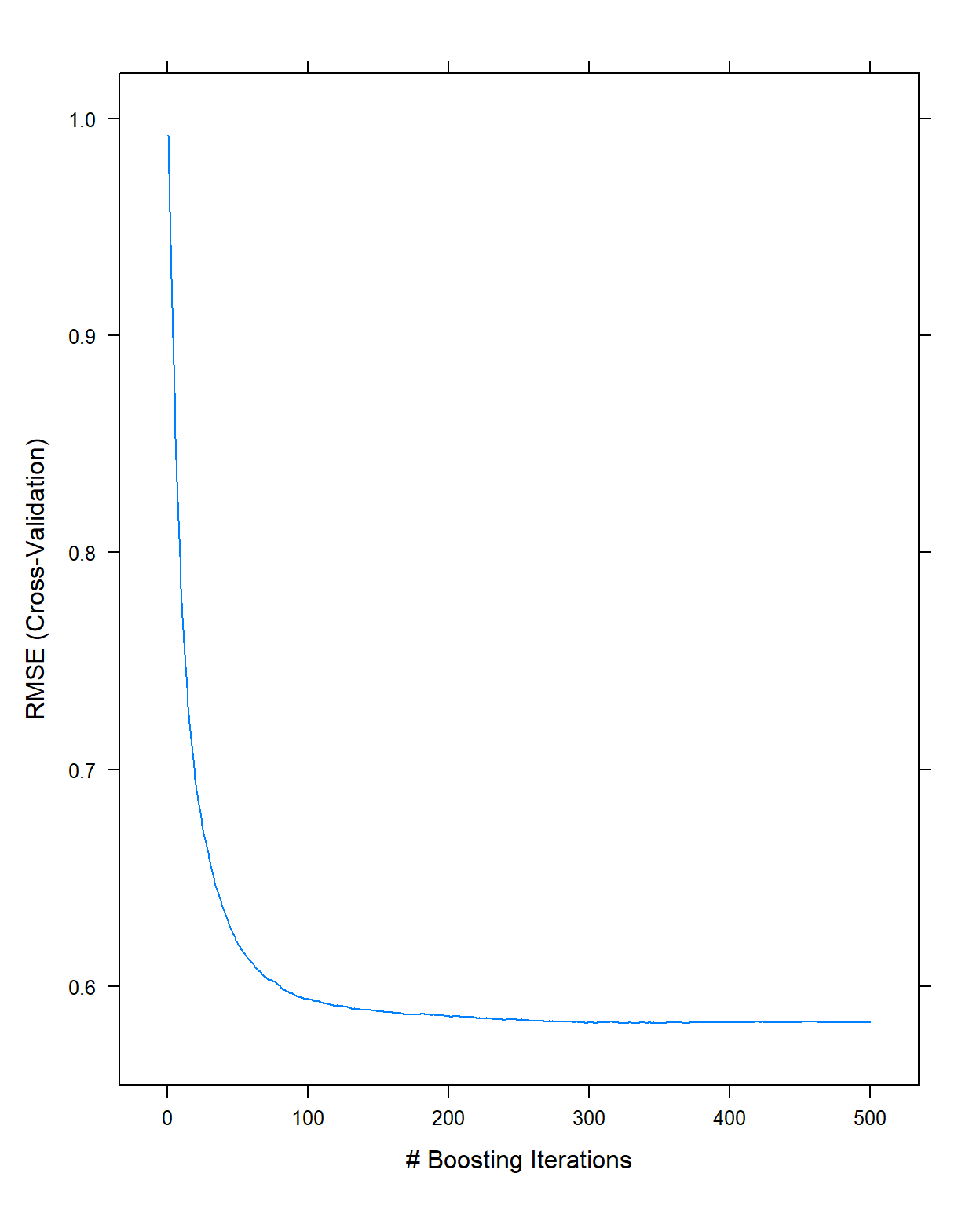It indicates there is not much improvement after 300 trees with these settings (this is just eyeballing, there is nothing specific about how to come up with this number). So, I will fix the number of trees to 300 for the next step.

Step 2: Tune the interaction depth and minimum number of observations

Now, we will fix the number of trees at 300 (n.trees = 300) and the learning rate at 0.1 (shrinkage=0.1).

Then, we will do a grid search by assigning values for the interaction depth from 1 to 15 and values for the minimum number of observations at 5, 10, 20, 30, 40, and 50. We still keep the bag fraction as 1.

grid <- expand.grid(shrinkage         = 0.1,
n.trees           = 300,
interaction.depth = 1:15,
n.minobsinnode    = c(5,10,20,30,40,50))

method    = 'gbm',
trControl = cv,
tuneGrid  = grid,
bag.fraction = 1,
verbose = FALSE)

gbm2$times $everything
user  system elapsed
87.53    1.55 7433.68

$final user system elapsed 75.17 0.14 75.30$prediction
 NA NA NA

This search took about 1 hour and 10 minutes. If we look at the cross-validates RMSE for all these 90 possible conditions, we see that the best result comes out when the interaction depth is equal to 9, and the minimum number of observations is equal to 50.

plot(gbm2,type='l')gbm2$bestTune  n.trees interaction.depth shrinkage n.minobsinnode 54 300 9 0.1 50 gbm2$results[which.min(gbm2$results$RMSE),]
   shrinkage interaction.depth n.minobsinnode n.trees      RMSE
54       0.1                 9             50     300 0.5759197
Rsquared       MAE     RMSESD RsquaredSD      MAESD
54 0.6904758 0.4582852 0.02670278 0.03351747 0.02441325

Step 3: Lower the learning rate and increase the number of trees

Now, we will fix the interaction depth at 9 (interaction.depth = 9) and the minimum number of observations at 50 (n.minobsinnode = 50). We will lower the learning rate to 0.01 (shrinkage=0.01) and increase the number of trees to 8000 (n.trees = 1:8000) to explore if a lower learning rate improves the performance.

grid <- expand.grid(shrinkage         = 0.01,
n.trees           = 1:8000,
interaction.depth = 9,
n.minobsinnode    = 50)

method    = 'gbm',
trControl = cv,
tuneGrid  = grid,
bag.fraction = 1,
verbose= FALSE)

gbm3$times $everything
user  system elapsed
1703.83    0.94 3821.78

$final user system elapsed 1689.69 0.09 1719.50$prediction
 NA NA NA
plot(gbm3,type='l')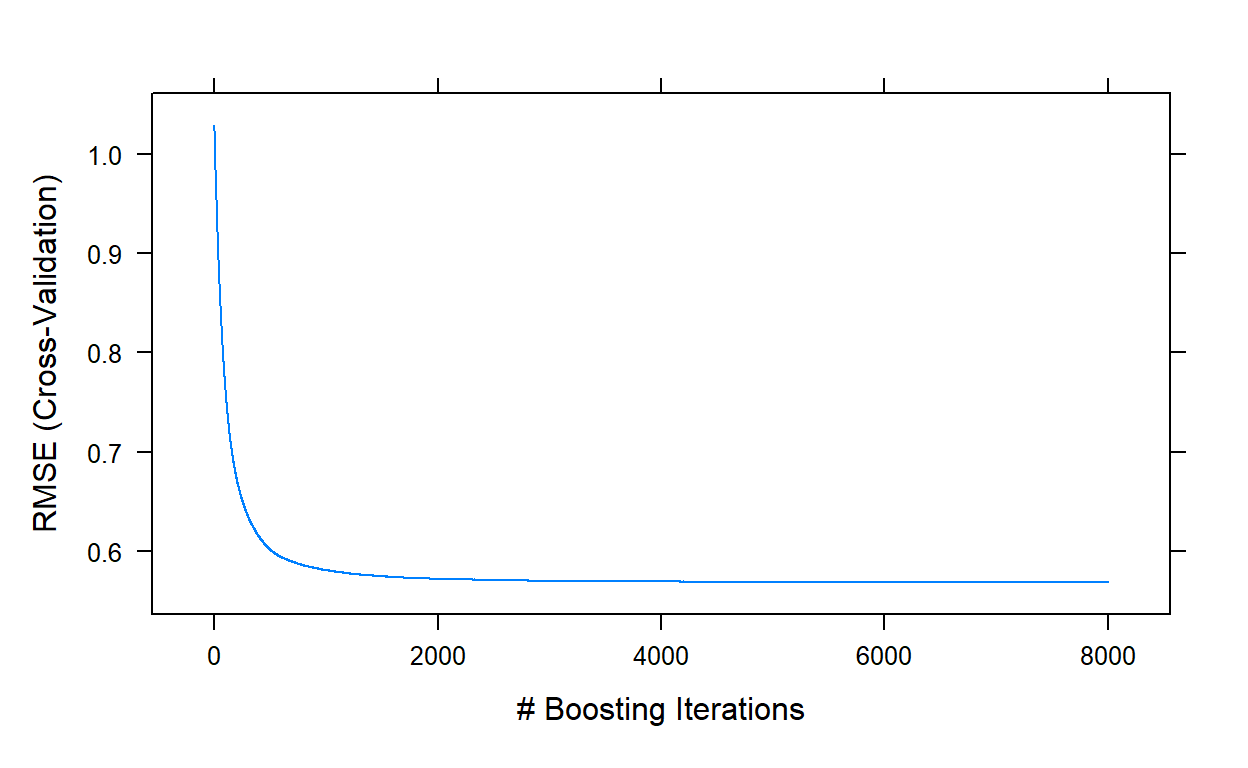gbm3$bestTune  n.trees interaction.depth shrinkage n.minobsinnode 7948 7948 9 0.01 50 gbm3$results[which.min(gbm3$results$RMSE),]
     shrinkage interaction.depth n.minobsinnode n.trees     RMSE
7948      0.01                 9             50    7948 0.568526
Rsquared      MAE     RMSESD RsquaredSD      MAESD
7948 0.6990292 0.453076 0.02218744 0.02806958 0.01829231

This run took about another 40 minutes. The best performance was obtained with a model of 7948 trees, and yielded an RMSE value of 0.5685. We can stop here and decide that this is our final model. Or, we can play with bag.fraction and see if we can improve the performance a little more.

Step 4: Tune Bag Fraction

To play with the bag.fraction, we should write our own syntax as caret::train does not allow it to be manipulated as a hyperparameter.

Notice that I fixed the values of shrinkage, n.trees,interaction.depth,n.minobsinnode at their optimal values.

Then, I write a for loop to iterate over different values of bag.fraction from 0.1 to 1 with increments of 0.05. I save the model object from each iteration in a list object.

grid <- expand.grid(shrinkage         = 0.01,
n.trees           = 7948,
interaction.depth = 9,
n.minobsinnode    = 50)

bag.fr <- seq(0.1,1,.05)

my.models <- vector('list',length(bag.fr))

for(i in 1:length(bag.fr)){

method    = 'gbm',
trControl = cv,
tuneGrid  = grid,
bag.fraction = bag.fr[i],
verbose= FALSE)
}

It took about 17 hours to complete with ten cores. Let’s check if it improved the performance.

cv.rmse <- c()

for(i in 1:length(bag.fr)){
cv.rmse[i] <- my.models[[i]]$results$RMSE
}

ggplot()+
geom_line(aes(x=bag.fr,y=cv.rmse))+
theme_bw()+
xlab('Bag Fraction')+
ylab('RMSE (Cross-validated)')+
scale_x_continuous(breaks = bag.fr)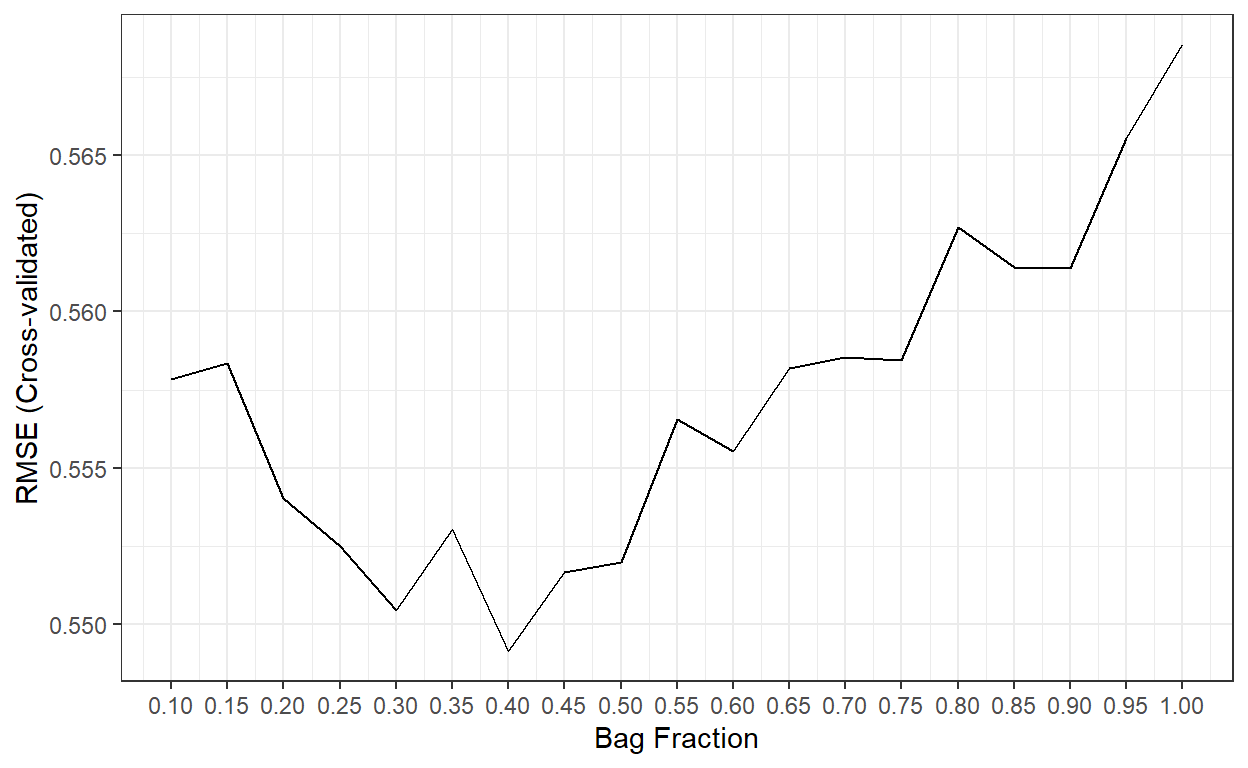The best performance was obtained when bag.fr is equal to 0.40.

Finally, we can check the performance of the final model with these settings on the test dataset and compare it to other methods.

final.gbm <- my.models[]

# Predictions from a Bagged tree model with 158 trees

# MAE

mean(abs(read_te$target - predicted_te))  0.4479047 # RMSE sqrt(mean((read_te$target - predicted_te)^2))
 0.5509891
# R-square

cor(read_te$target,predicted_te)^2  0.7135852 R-square MAE RMSE Ridge Regression 0.727 0.435 0.536 Lasso Regression 0.725 0.434 0.538 Gradient Boosting 0.714 0.448 0.551 Random Forests 0.671 0.471 0.596 Bagged Trees 0.656 0.481 0.604 Linear Regression 0.644 0.522 0.644 KNN 0.623 0.500 0.629 Decision Tree 0.497 0.577 0.729 # 6. Predicting Recidivism Using Gradient Boosting Trees The code below implements a similar strategy and demonstrates how to fit a Gradient Boosting Tree model for the Recidivism dataset to predict recidivism in Year 2. Import the dataset and initial data preparation # Import data recidivism <- read.csv(here('data/recidivism_y1 removed and recoded.csv'), header=TRUE) # List of variable types in the dataset outcome <- c('Recidivism_Arrest_Year2') id <- c('ID') categorical <- c('Residence_PUMA', 'Prison_Offense', 'Age_at_Release', 'Supervision_Level_First', 'Education_Level', 'Prison_Years', 'Gender', 'Race', 'Gang_Affiliated', 'Prior_Arrest_Episodes_DVCharges', 'Prior_Arrest_Episodes_GunCharges', 'Prior_Conviction_Episodes_Viol', 'Prior_Conviction_Episodes_PPViolationCharges', 'Prior_Conviction_Episodes_DomesticViolenceCharges', 'Prior_Conviction_Episodes_GunCharges', 'Prior_Revocations_Parole', 'Prior_Revocations_Probation', 'Condition_MH_SA', 'Condition_Cog_Ed', 'Condition_Other', 'Violations_ElectronicMonitoring', 'Violations_Instruction', 'Violations_FailToReport', 'Violations_MoveWithoutPermission', 'Employment_Exempt') numeric <- c('Supervision_Risk_Score_First', 'Dependents', 'Prior_Arrest_Episodes_Felony', 'Prior_Arrest_Episodes_Misd', 'Prior_Arrest_Episodes_Violent', 'Prior_Arrest_Episodes_Property', 'Prior_Arrest_Episodes_Drug', 'Prior_Arrest_Episodes_PPViolationCharges', 'Prior_Conviction_Episodes_Felony', 'Prior_Conviction_Episodes_Misd', 'Prior_Conviction_Episodes_Prop', 'Prior_Conviction_Episodes_Drug', 'Delinquency_Reports', 'Program_Attendances', 'Program_UnexcusedAbsences', 'Residence_Changes', 'Avg_Days_per_DrugTest', 'Jobs_Per_Year') props <- c('DrugTests_THC_Positive', 'DrugTests_Cocaine_Positive', 'DrugTests_Meth_Positive', 'DrugTests_Other_Positive', 'Percent_Days_Employed') # Convert all nominal, ordinal, and binary variables to factors for(i in categorical){ recidivism[,i] <- as.factor(recidivism[,i]) } # Write the recipe require(recipes) blueprint_recidivism <- recipe(x = recidivism, vars = c(categorical,numeric,props,outcome,id), roles = c(rep('predictor',48),'outcome','ID')) %>% step_indicate_na(all_of(categorical),all_of(numeric),all_of(props)) %>% step_zv(all_numeric()) %>% step_impute_mean(all_of(numeric),all_of(props)) %>% step_impute_mode(all_of(categorical)) %>% step_logit(all_of(props),offset=.001) %>% step_poly(all_of(numeric),all_of(props),degree=2) %>% step_normalize(paste0(numeric,'_poly_1'), paste0(numeric,'_poly_2'), paste0(props,'_poly_1'), paste0(props,'_poly_2')) %>% step_dummy(all_of(categorical),one_hot=TRUE) %>% step_num2factor(Recidivism_Arrest_Year2, transform = function(x) x + 1, levels=c('No','Yes')) blueprint_recidivism Train/Test Split and Cross-validation Settings # Train/Test Split loc <- which(recidivism$Training_Sample==1)

recidivism_tr  <- recidivism[loc, ]
recidivism_te  <- recidivism[-loc, ]

# Cross validation settings

set.seed(10302021) # for reproducibility

recidivism_tr = recidivism_tr[sample(nrow(recidivism_tr)),]

# Create 10 folds with equal size

folds = cut(seq(1,nrow(recidivism_tr)),breaks=10,labels=FALSE)

# Create the list for each fold

my.indices <- vector('list',10)
for(i in 1:10){
my.indices[[i]] <- which(folds!=i)
}

cv <- trainControl(method = "cv",
index  = my.indices,
classProbs = TRUE,
summaryFunction = mnLogLoss)

Step 1: Initial model fit to tune the number of trees

We fix the learning rate at 0.1 (shrinkage=0.1), interaction depth at 5 (interaction.depth=5), and the minimum number of observations at 10 (n.minobsinnode = 10). We do a grid search for the optimal number of trees from 1 to 1000 (n.trees = 1:1000).

grid <- expand.grid(shrinkage         = 0.1,
n.trees           = 1:1000,
interaction.depth = 5,
n.minobsinnode    = 10)

gbm1 <- caret::train(blueprint_recidivism,
data         = recidivism_tr,
method       = 'gbm',
trControl    = cv,
tuneGrid     = grid,
bag.fraction = 1,
metric       = 'logLoss')

plot(gbm1,type='l')

gbm1$bestTune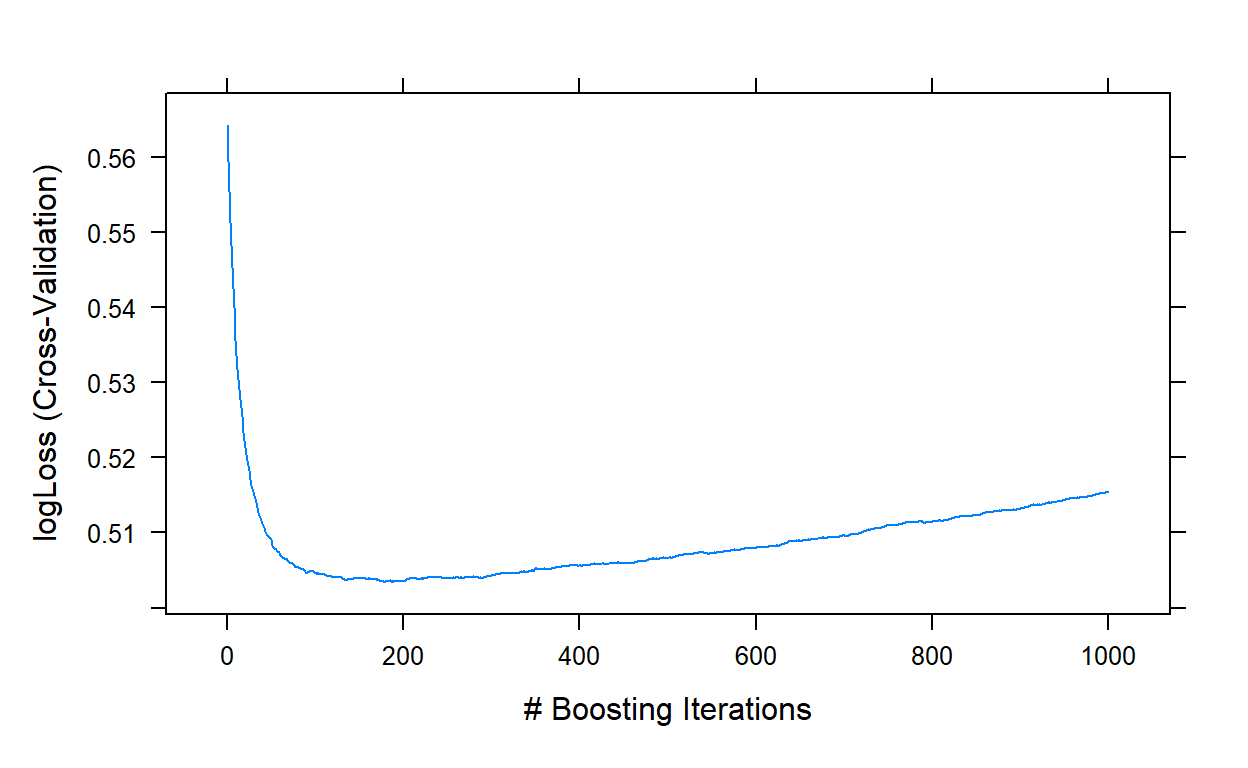n.trees interaction.depth shrinkage n.minobsinnode 178 178 5 0.1 10 It indicates that a model with 178 trees is optimal at the initial search. Step 2: Tune the interaction depth and minimum number of observations We fix the number of trees at 178 (n.trees = 178) and the learning rate at 0.1 (shrinkage=0.1). Then, we do a grid search by assigning values for the interaction depth from 1 to 15 and the minimum number of observations at 5, 10, 20, 30, 40, and 50. grid <- expand.grid(shrinkage = 0.1, n.trees = 178, interaction.depth = 1:15, n.minobsinnode = c(5,10,20,30,40,50)) gbm2 <- caret::train(blueprint_recidivism, data = recidivism_tr, method = 'gbm', trControl = cv, tuneGrid = grid, bag.fraction = 1, metric = 'logLoss') plot(gbm2) gbm2$bestTune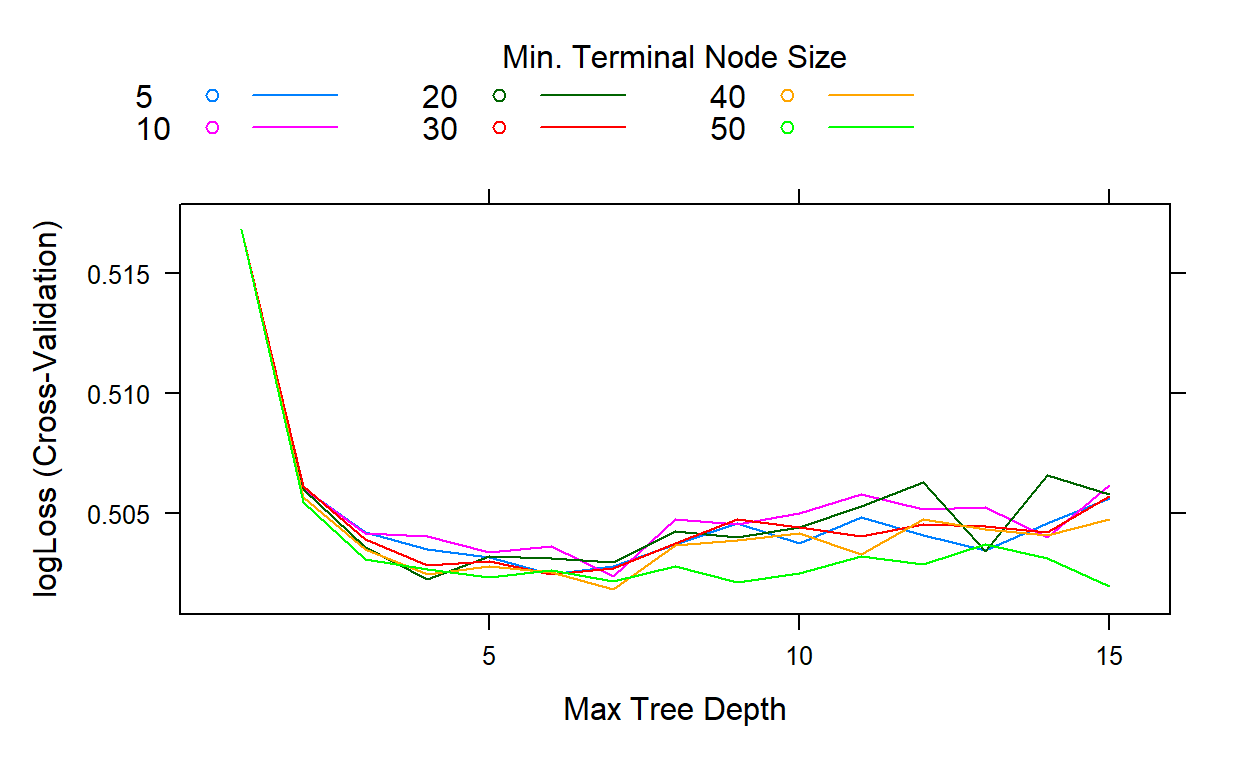n.trees interaction.depth shrinkage n.minobsinnode
41     178                 7       0.1             40

The search indicates that the best performance is obtained when the interaction depth equals 7 and the minimum number of observations equals 40.

Step 3: Lower the learning rate and increase the number of trees

We fix the interaction depth at 7 (interaction.depth = 4) and the minimum number of observations at 40 (n.minobsinnode = 40). We will lower the learning rate to 0.01 (shrinkage=0.01) and increase the number of trees to 10000 (n.trees = 1:10000) to explore if a lower learning rate improves the performance.

grid <- expand.grid(shrinkage         = 0.01,
n.trees           = 1:10000,
interaction.depth = 7,
n.minobsinnode    = 40)

gbm3 <- caret::train(blueprint_recidivism,
data         = recidivism_tr,
method       = 'gbm',
trControl    = cv,
tuneGrid     = grid,
bag.fraction = 1,
metric       = 'logLoss')

plot(gbm3,type='l')

gbm3$bestTunen.trees interaction.depth shrinkage n.minobsinnode 1731 1731 7 0.01 40 Step 4: Tune the bag fraction grid <- expand.grid(shrinkage = 0.01, n.trees = 1731, interaction.depth = 7, n.minobsinnode = 40) bag.fr <- seq(0.1,1,.05) my.models <- vector('list',length(bag.fr)) for(i in 1:length(bag.fr)){ my.models[[i]] <- caret::train(blueprint_recidivism, data = recidivism_tr, method = 'gbm', trControl = cv, tuneGrid = grid, bag.fraction = bag.fr[i]) } cv.LogL <- c() for(i in 1:length(bag.fr)){ cv.LogL[i] <- my.models[[i]]$results$logLoss } ggplot()+ geom_line(aes(x=bag.fr,y=cv.LogL))+ theme_bw()+ xlab('Bag Fraction')+ ylab('LogLoss (Cross-validated)')+ scale_x_continuous(breaks = bag.fr)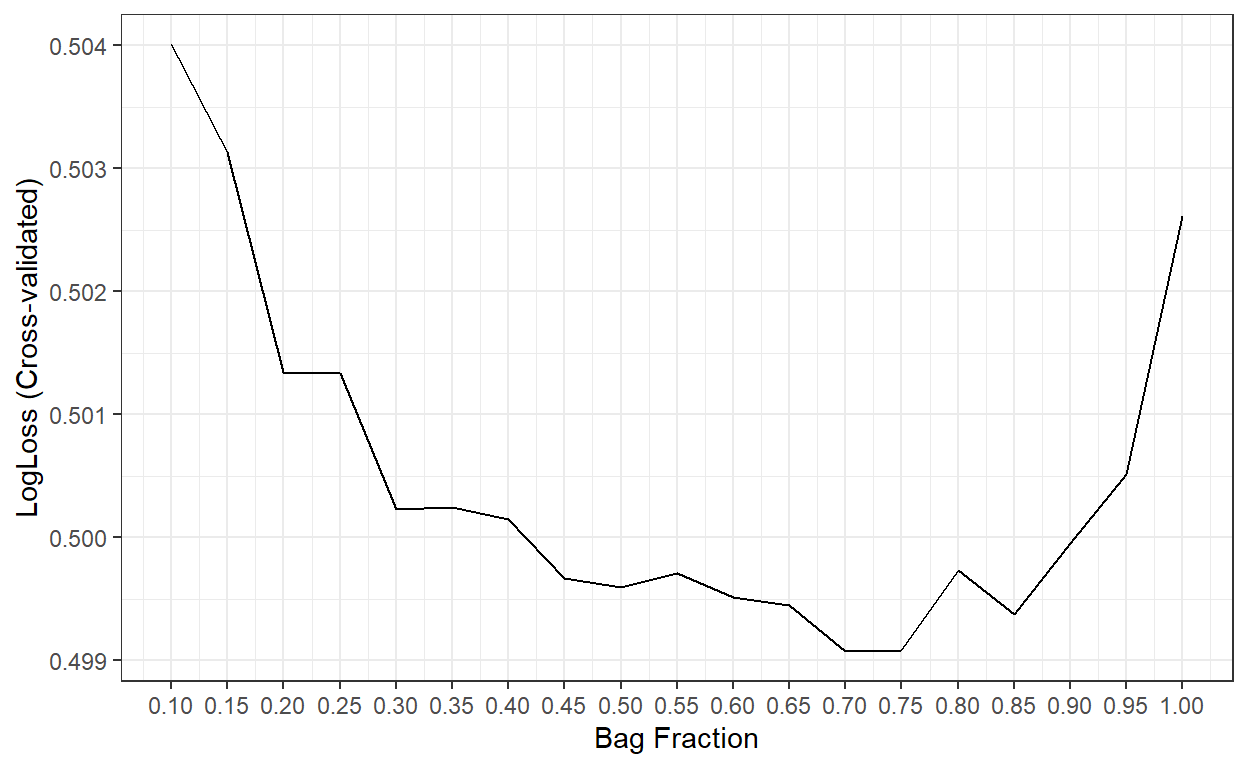bag.fr[which.min(cv.LogL)]  0.7 The best result is obtained when the bag fraction is 0.7. So, we will proceed with that as our final model. Step 5: Final Predictions on Test Set final.gbm <- my.models[] # Predict the probabilities for the observations in the test dataset predicted_te <- predict(final.gbm, recidivism_te, type='prob') head(predicted_te)  No Yes 1 0.9479557 0.05204431 2 0.6394094 0.36059065 3 0.7002056 0.29979444 4 0.6725847 0.32741529 5 0.8364610 0.16353899 6 0.7666956 0.23330437 # Compute the AUC require(cutpointr) cut.obj <- cutpointr(x = predicted_te$Yes,
class = recidivism_te$Recidivism_Arrest_Year2) auc(cut.obj)  0.7465408 # Confusion matrix assuming the threshold is 0.5 pred_class <- ifelse(predicted_te$Yes>.5,1,0)

confusion <- table(recidivism_te\$Recidivism_Arrest_Year2,pred_class)

confusion
   pred_class
0    1
0 3957  189
1 1109  205
# True Negative Rate

confusion[1,1]/(confusion[1,1]+confusion[1,2])
 0.9544139
# False Positive Rate

confusion[1,2]/(confusion[1,1]+confusion[1,2])
 0.04558611
# True Positive Rate

confusion[2,2]/(confusion[2,1]+confusion[2,2])
 0.1560122
# Precision

confusion[2,2]/(confusion[1,2]+confusion[2,2])
 0.5203046

Comparison of Results

-LL AUC ACC TPR TNR FPR PRE
Gradient Boosting Trees 0.499 0.747 0.763 0.156 0.954 0.046 0.520
Random Forests 0.507 0.725 0.761 0.153 0.954 0.046 0.514
Bagged Trees 0.506 0.724 0.759 0.144 0.954 0.046 0.500
Logistic Regression 0.510 0.719 0.755 0.142 0.949 0.051 0.471
Logistic Regression with Ridge Penalty 0.511 0.718 0.754 0.123 0.954 0.046 0.461
Logistic Regression with Lasso Penalty 0.509 0.720 0.754 0.127 0.952 0.048 0.458
Logistic Regression with Elastic Net 0.509 0.720 0.753 0.127 0.952 0.048 0.456
KNN ? ? ? ? ? ? ?
Decision Tree 0.558 0.603 0.757 0.031 0.986 0.014 0.423

### Reuse

Text and figures are licensed under Creative Commons Attribution CC BY 4.0. The figures that have been reused from other sources don't fall under this license and can be recognized by a note in their caption: "Figure from ...".